# Excel Relative References

## Relative and Absolute References

Cells in Excel have unique references, which is its location.

References are used in formulas to do calculations, and the fill function can be used to continue formulas sidewards, downwards and upwards.

Excel has two types of references:

1. Relative references
2. Absolute references

Absolute reference is a choice we make. It is a command which tells Excel to lock a reference.

The dollar sign (`\$`) is used to make references absolute.

Example of relative reference: `A1`

Example of absolute reference: `\$A\$1`

## Relative reference

References are relative by default, and are without dollar sign (\$).

The relative reference makes the cells reference free. It gives the fill function freedom to continue the order without restrictions.

Let's have a look at a relative reference example, helping the Pokemon trainers to count their Pokeballs (`B2:B7`) and Great balls (`C2:C7`).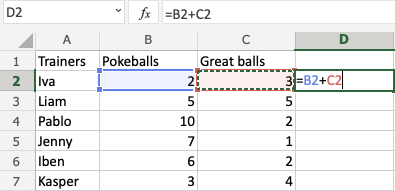Copy Values

The result is: `D2(5)`:Next, fill the range `D2:D7`: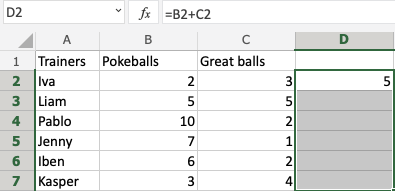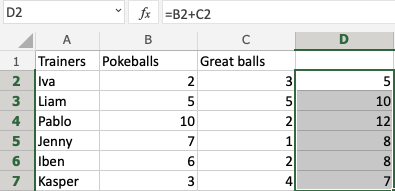The references being relative allows the fill function to continue the formula for rows downwards.

Have a look at the formulas in `D2:D7`. Notice that it calculates the next row as you fill.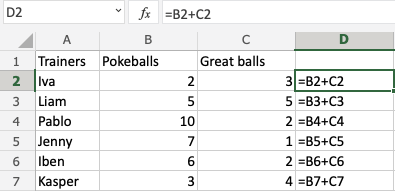## A Non-Working Example

Let's try an example that will not work.

Fill `D2:G2`, filling to the right instead of downwards. Resulting in strange numbers: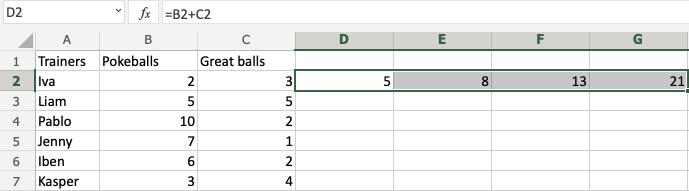Have a look at the formulas.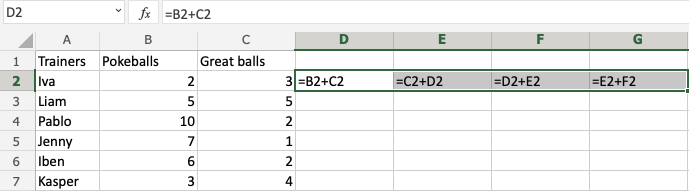It assumes that we are calculating sidewards and not downwards.

The numbers that we want to calculate need to be in the same direction as we fill.

## Excel Exercise:

Type reference `B2` as relative:

```
```

Start the Exercise